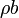# Errata: In Equation (3.19), the second term in the window dynamics should contain \rho c

In equation (3.19), the dynamics for the window size contain a termwhich should beThe correct equation is